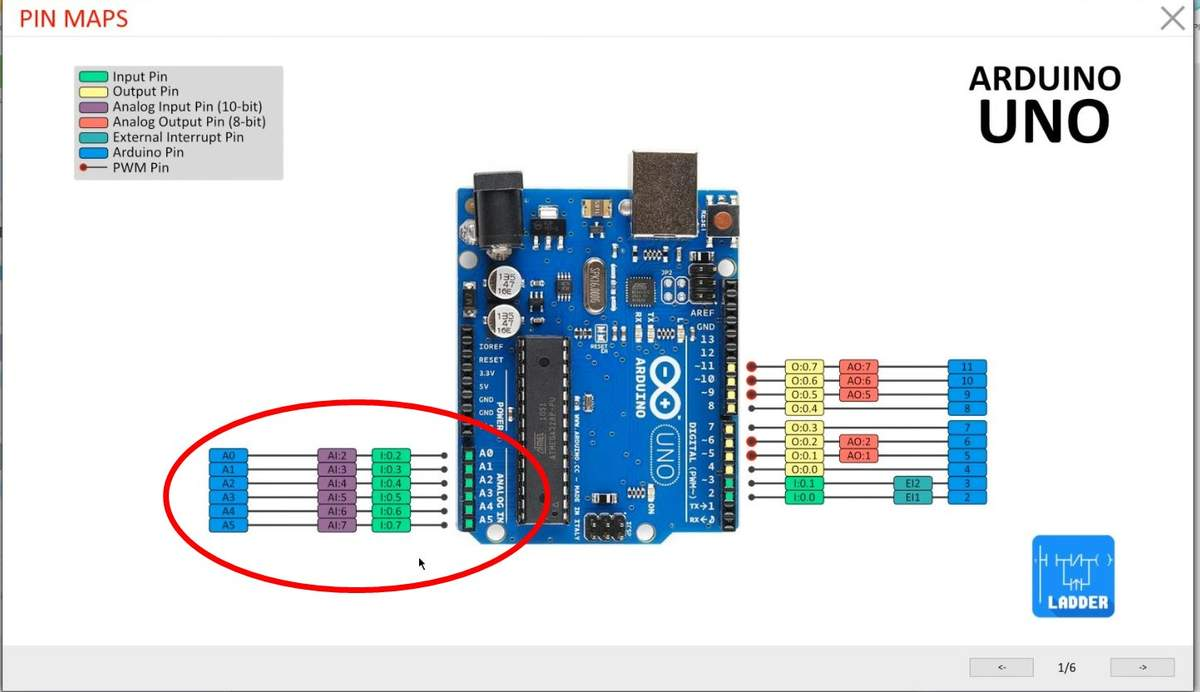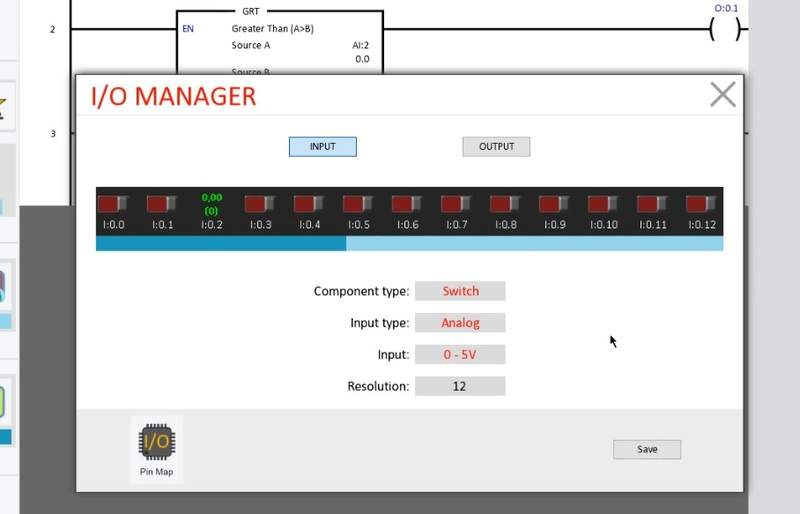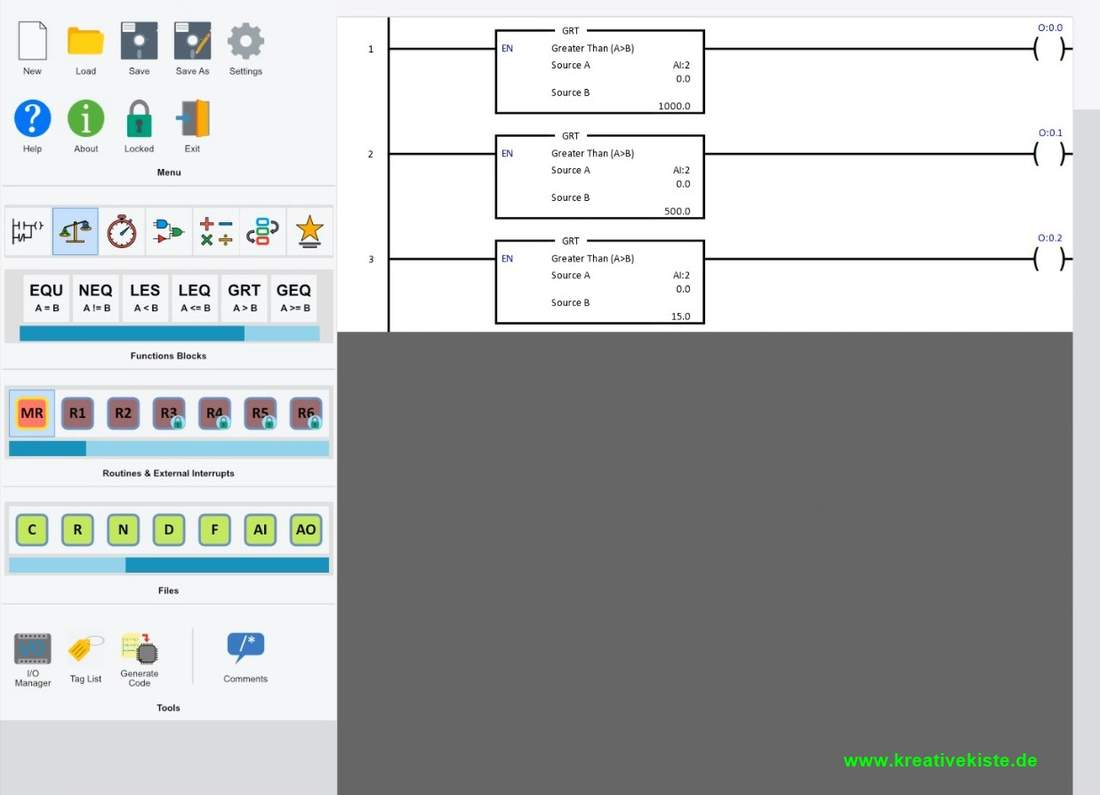# Arduino PLC Ladder Editor, greater and less than

Greater than and smaller than are some of the most important functions for the analog inputs. Because with greater and less than you can tell a regulation and / or control whether a value is OK or not. Technically correct, one speaks of a fault that triggers a control process. The FAULT is a deviation from the target value and we can determine this deviation with a greater than and less than function.

As a rule, the open brackets are used as a symbol or sign for this function. Remember: a hungry crocodile always wants to eat a lot. The open mouth therefore points to the larger number.

If only the functions larger and smaller than are used, certain malfunctions cannot be correctly regulated, which is why there are some other comparison functions.

 TRUE if the first number is less than the second: < TRUE if the first number is less than the second > TRUE if the first number is equal to or less than the second =< TRUE if the first number is equal to or greater than the second => TRUE if both numbers are the same == TRUE if both numbers are not the same !=

"Plcladdersimulator2" also has these various functions that enable regulation.

As a test, I connected 3 LEDs with outputs 4 (0.0), 5 (0.1) and 6 (0.2) and connected a potentiometer between GND and VCC to the analog input A0.The analog PINs are marked purple, as you can see in the software's "PIN MAP".Since the analog PINs can also be used as digital ones, you must first switch from digital to analog. It still has to be set whether the VCC PIN of the potentiometer of 3.3 or 5 volts is used. The remaining settings should not be changed.If you add a "greater or less than" block, a dialog window for the function opens immediately.

• DN, the output is at "TRUE" or greater or less than is switched
• SourceA, is the first value of the function which is then compared with SourceB or Constant. the analog input can be selected here. Since I0 and I1 are purely digital PINs (Arduino PIN 2 and 3), the analog PIN is A0 on the Arduino, the AI: 2 in the software.
• SourceB, here a second analog input can be selected as a comparison for larger or smaller.
• Constant: if you want to compare with a fixed value, then enter a number here and leave SourceB free.

Now we compare AI2 with the fixed value 1000.0. If the value at AI: 2 is greater than 1000, B0: 0 "HIGH" is switched on.In this way, different values can be compared and, depending on whether the outputs are switched or "flags" are set. In this case the LEDs at the outputs would turn on or go out one after the other when the potentiometer is turned.If the "less" functions are used instead of the "greater", the LEDs go ON and OFF in reverse order when the potentiometer is turned. Since the conditions are not met if the value is greater than X, but rather if the value is less than X.

The simulation does not completely agree with the program, in the simulation the value goes much higher at 5 volts. In my experiments, the value at 5 volts only goes up to the "known" 1023. Therefore set the values between 0 and 1023.If only one LED is to be "HIGH" at a time, the others can be switched off via a contact. In this way, well-functioning regulations and controls can be programmed very easily.### Arduino Kontaktplan

Wir nutzen Cookies auf unserer Website. Einige von ihnen sind essenziell für den Betrieb der Seite, während andere uns helfen, diese Website und die Nutzererfahrung zu verbessern (Tracking Cookies). Sie können selbst entscheiden, ob Sie die Cookies zulassen möchten. Bitte beachten Sie, dass bei einer Ablehnung womöglich nicht mehr alle Funktionalitäten der Seite zur Verfügung stehen.